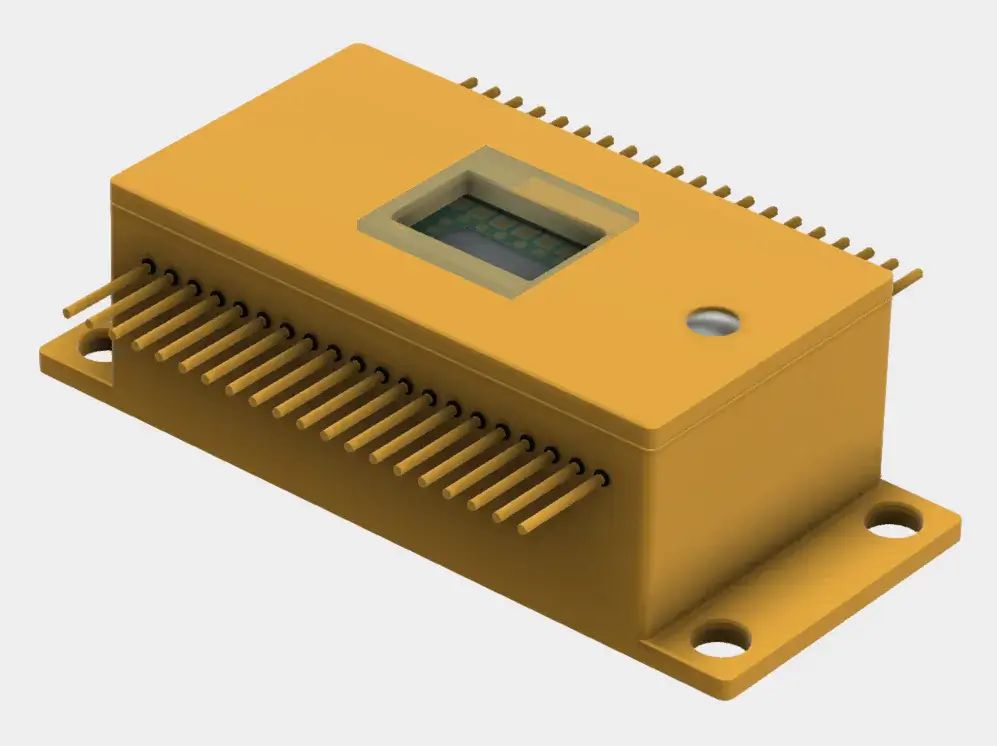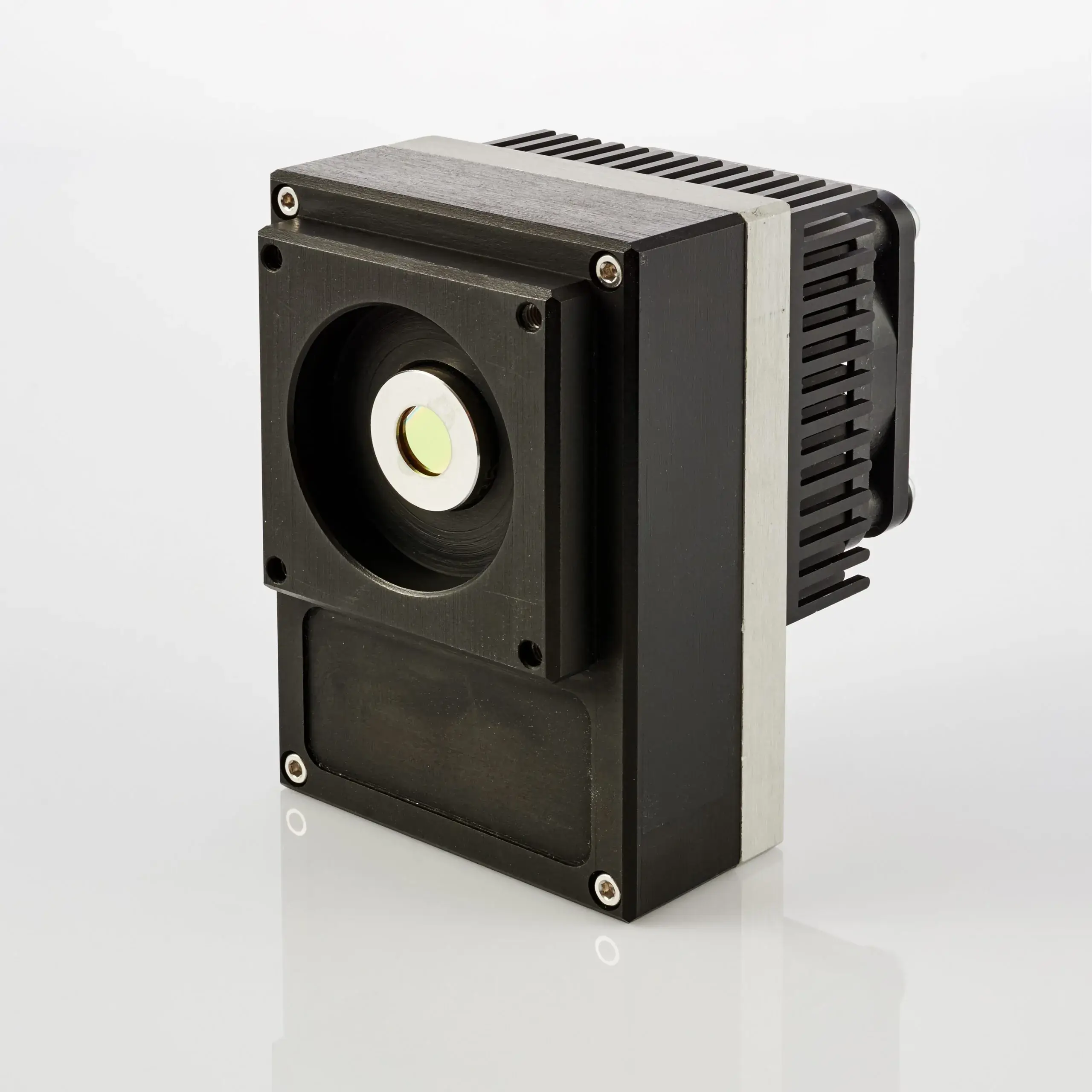# HgCdTe (MCT) Multi Channel Detection Modules

VIGO HgCdTe (MCT) multi channel detection modules are ideal for gas analysis, measuring the movement of a beam, the distance traveled, or as feedback for alignment systems.

### Filter resultsImage

Name

Optimum wavelength

?

λopt, µm

Active area

?

A, mm×mm

Detectivity

?

D* (λopt), cm⋅Hz1/2/W

Output voltage responsivity

?

Rvopt, Rload = 50 Ω), V/W

-3dB Bandwidth

Datasheet### 32EM-5-01

Wavelength

5.0

Active area

32×(0.125×1)

Detectivity

≥ 2.2×10$$^{9}$$

Output voltage

≥ 3.5×10$$^{4}$$

Bandwidth

DC to ≥ 400 kHz### 32EM-5-02

Wavelength

5.0

Active area

32×(0.1×0.1)

Detectivity

≥ 2.2×10$$^{9}$$

Output voltage

≥ 4.6×10$$^{4}$$

Bandwidth

DC to ≥ 650 kHz### 4EM-5

Wavelength

5

Active area

Detectivity

≥ 6.8×10$$^{9}$$

Output voltage

≥ 1.6×10$$^{5}$$

Bandwidth

DC to ≥ 1 MHz### QM-5

Wavelength

5

Active area

Detectivity

≥ 6.8×10$$^{9}$$

Output voltage

≥ 1.6×10$$^{5}$$

Bandwidth

DC to ≥ 1 MHz### QM-10.6

Wavelength

10.6

Active area

Detectivity

≥ 4.5×10$$^{6}$$

Output voltage

≥ 1.1×10$$^{2}$$

Bandwidth

DC to ≥ 1 MHz